Email us to get an instant 20% discount on highly effective K-12 Math & English kwizNET Programs!

#### Online Quiz (WorksheetABCD)

Questions Per Quiz = 2 4 6 8 10

### High School Mathematics - 28.4 Theorems - Circles

 Theorem: Angle is a semi circle is a right angle.Given : Angle AOB is in semi circle. To prove: Angle ACB = 90o Proof: Angle ACB = 1/2 angle AOB (angle subtended at the centre is twice angle at circumference) Angle AOB = 180o (straight line) Hence ACB = 90o Theorem: Angles in the same segment of a circle are equal.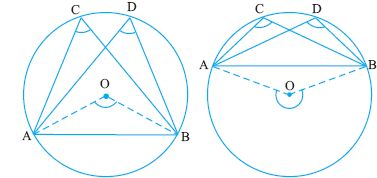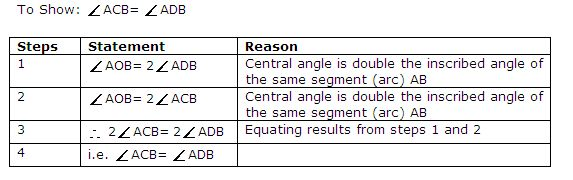Directions: Solve the following
 Q 1: ABCD is a cyclic quadrilateral and BC = CD. Show that AC bisects �BAD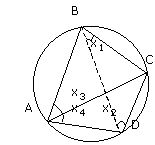Answer: Q 2: In the figure, PAQ and RAS are straight lines. Show that angPXR = angQYS.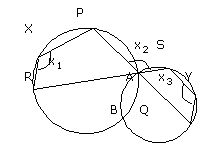Answer: Q 3: If diagonals AC and BD of a quadrilateral intersect at M. Prove that a line drawn through M to bisect any side of the quadrilateral is perpendicular to the opposite side.Answer: Q 4: find x.90 degrees110 degrees55 degrees Q 5: In the figure, CAD and CBE are straight lines. If CA is the diameter of the circle ABC, find angle ADE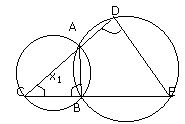45 degrees180 degrees90 degrees Q 6: Angles in a semicircle is always ____.270 degrees90 degrees180 degrees Q 7: Find x.35 degrees140 degrees70 degrees Q 8: Find the angle x.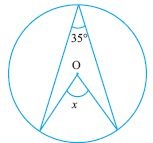17.5 degrees70 degrees90 degrees Question 9: This question is available to subscribers only! Question 10: This question is available to subscribers only!

#### Subscription to kwizNET Learning System offers the following benefits:

• Unrestricted access to grade appropriate lessons, quizzes, & printable worksheets
• Instant scoring of online quizzes
• Progress tracking and award certificates to keep your student motivated
• Unlimited practice with auto-generated 'WIZ MATH' quizzes